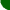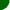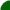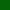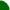welcome/ java-mcmc/ software/ papers/ links/ email mesitemap software essays mathematics unix links

Coupling constructions for Markov chains are closely related to the study of convergence to the stationary distribution and so called mixing times. On this page, I shall discuss briefly a universal coupling method which applies to any Markov chain whose transition probabilities are known. This includes all Metropolis-Hastings samplers used in practice. An accompanying paper may be found here (ps/pdf).

Let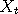and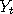be two Markov chains with the same transition density. A common way of simulating such chains is by the rules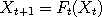and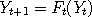, where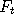refers to some (non unique) computer program which implements one simulation step (iteration) at time. It is exceedingly unlikely that, for a typical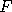, we can get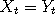, at any time. Why should we look for ways of simulating chains which can couple in a finite time? Theory tells us that if we wait until two suitably chosen chains meet, then we have waited long enough for proper mixing. More precisely, the coupling inequality says that, if the Y process is stationary, then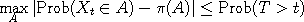where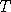is the first time thatoccurs, andis the stationary distribution. In effect, the two chains have lost all dependence upon their initial positions, which is useful for Markov chain Monte Carlo. Moreover, knowing how to make Markov chains come together is a fundamental ingredient of perfect simulation. One easy way to construct coalescing Markov chains consists in modifying an existing transition rulein such a way that nearby chains have a chance of coming together in one step, the transition densityis preserved.

Such a rule is described in the accompagnying paper (ps/pdf), but can also be described briefly in the following words. At each time t, we select at random a point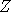in the state space, called a catalyst. Next, rather than update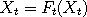(and similarly for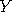), we let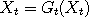(and similarly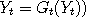, where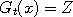with a suitably chosen probability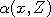and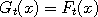otherwise. The right choice for a means that we do not destroy the transition probabilities, and if both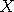andsimultaneously accept the move tothen they must meet. The coupling method outlined above does not just work for two chains, but can be applied to as many processes as desired simultaneously. Moreover, as many catalysts as desired can be introduced, since the transition density is preserved every time. The applet below illustrates this in the case when the underlying chains are Metropolized random walks with Gaussian increments (as seen in M-H algorithms) and the catalysts are chosen uniformly in the unit square. To distinguish the catalysts from the underlying chains, the former are surrounded by circles. You can choose the number of catalysts as well as the number of Markov chains to couple, together with the corresponding jump sizes denoted s. If you select zero catalysts, you see that the chains come closer together but do not meet eventually (except due to numerical errors). If you click once inside the image, the simulation is paused. Clicking again resumes it.

To view this applet, please install the Java Plugin.
 Delay Number of chains chainNumber of catalysts

An interesting question to ponder here is the following: what is the optimal number of catalysts to ensure that all test chains coalesce in as few steps as possible? Theory tells us that it doesn't matter how many catalysts we use, since in each case we get coalescence in a finite time. However, a bit of experimentation might convince you that, firstly, two catalysts are better than one, and secondly, a great many catalysts just makes things worse. This last statement can be backed up by theory, see

 sitemap software essays mathematics unix links